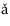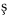# 13.4: Discussion and Exercises

$$\newcommand{\vecs}{\overset { \rightharpoonup} {\mathbf{#1}} }$$ $$\newcommand{\vecd}{\overset{-\!-\!\rightharpoonup}{\vphantom{a}\smash {#1}}}$$$$\newcommand{\id}{\mathrm{id}}$$ $$\newcommand{\Span}{\mathrm{span}}$$ $$\newcommand{\kernel}{\mathrm{null}\,}$$ $$\newcommand{\range}{\mathrm{range}\,}$$ $$\newcommand{\RealPart}{\mathrm{Re}}$$ $$\newcommand{\ImaginaryPart}{\mathrm{Im}}$$ $$\newcommand{\Argument}{\mathrm{Arg}}$$ $$\newcommand{\norm}{\| #1 \|}$$ $$\newcommand{\inner}{\langle #1, #2 \rangle}$$ $$\newcommand{\Span}{\mathrm{span}}$$ $$\newcommand{\id}{\mathrm{id}}$$ $$\newcommand{\Span}{\mathrm{span}}$$ $$\newcommand{\kernel}{\mathrm{null}\,}$$ $$\newcommand{\range}{\mathrm{range}\,}$$ $$\newcommand{\RealPart}{\mathrm{Re}}$$ $$\newcommand{\ImaginaryPart}{\mathrm{Im}}$$ $$\newcommand{\Argument}{\mathrm{Arg}}$$ $$\newcommand{\norm}{\| #1 \|}$$ $$\newcommand{\inner}{\langle #1, #2 \rangle}$$ $$\newcommand{\Span}{\mathrm{span}}$$$$\newcommand{\AA}{\unicode[.8,0]{x212B}}$$

The first data structure to provide $$O(\log\mathtt{w})$$ time $$\mathtt{add(x)}$$, $$\mathtt{remove(x)}$$, and $$\mathtt{find(x)}$$ operations was proposed by van Emde Boas and has since become known as the van Emde Boas (or stratified) tree . The original van Emde Boas structure had size $$2^{\mathtt{w}}$$, making it impractical for large integers.

The XFastTrie and YFastTrie data structures were discovered by Willard . The XFastTrie structure is closely related to van Emde Boas trees; for instance, the hash tables in an XFastTrie replace arrays in a van Emde Boas tree. That is, instead of storing the hash table $$\mathtt{t[i]}$$, a van Emde Boas tree stores an array of length $$2^{\mathtt{i}}$$.

Another structure for storing integers is Fredman and Willard's fusion trees . This structure can store $$\mathtt{n}$$ $$\mathtt{w}$$-bit integers in $$O(\mathtt{n})$$ space so that the $$\mathtt{find(x)}$$ operation runs in $$O((\log \mathtt{n})/(\log\mathtt{w}))$$ time. By using a fusion tree when $$\log \mathtt{w} > \sqrt{\log \mathtt{n}}$$ and a YFastTrie when $$\log \mathtt{w} \le \sqrt{\log \mathtt{n}}$$, one obtains an $$O(\mathtt{n})$$ space data structure that can implement the $$\mathtt{find(x)}$$ operation in $$O(\sqrt{\log \mathtt{n}})$$ time. Recent lower-bound results of Ptracu and Thorup  show that these results are more or less optimal, at least for structures that use only $$O(\mathtt{n})$$ space.

Exercise $$\PageIndex{1}$$

Design and implement a simplified version of a BinaryTrie that does not have a linked list or jump pointers, but for which $$\mathtt{find(x)}$$ still runs in $$O(\mathtt{w})$$ time.

Exercise $$\PageIndex{2}$$

Design and implement a simplified implementation of an XFastTrie that doesn't use a binary trie at all. Instead, your implementation should store everything in a doubly-linked list and $$\mathtt{w}+1$$ hash tables.

Exercise $$\PageIndex{3}$$

We can think of a BinaryTrie as a structure that stores bit strings of length $$\mathtt{w}$$ in such a way that each bitstring is represented as a root to leaf path. Extend this idea into an SSet implementation that stores variable-length strings and implements $$\mathtt{add(s)}$$, $$\mathtt{remove(s)}$$, and $$\mathtt{find(s)}$$ in time proporitional to the length of $$\mathtt{s}$$.

Hint: Each node in your data structure should store a hash table that is indexed by character values.

Exercise $$\PageIndex{4}$$

For an integer $$\mathtt{x}\in\{0,\ldots2^{\mathtt{w}}-1\}$$, let $$d(\mathtt{x})$$ denote the difference between $$\mathtt{x}$$ and the value returned by $$\mathtt{find(x)}$$ [if $$\mathtt{find(x)}$$ returns $$\mathtt{null}$$, then define $$d(\mathtt{x})$$ as $$2^\mathtt{w}$$]. For example, if $$\mathtt{find(23)}$$ returns 43, then $$d(23)=20$$.

1. Design and implement a modified version of the $$\mathtt{find(x)}$$ operation in an XFastTrie that runs in $$O(1+\log d(\mathtt{x}))$$ expected time. Hint: The hash table $$t[\mathtt{w}]$$ contains all the values, $$\mathtt{x}$$, such that $$d(\mathtt{x})=0$$, so that would be a good place to start.
2. Design and implement a modified version of the $$\mathtt{find(x)}$$ operation in an XFastTrie that runs in $$O(1+\log\log d(\mathtt{x}))$$ expected time.

This page titled 13.4: Discussion and Exercises is shared under a CC BY license and was authored, remixed, and/or curated by Pat Morin (Athabasca University Press) .